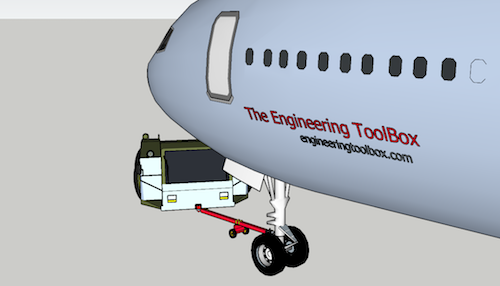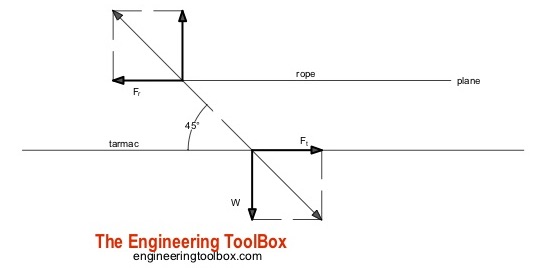Engineering ToolBox - Resources, Tools and Basic Information for Engineering and Design of Technical Applications!

# Pulling an Airplane

## Force required to pull an air plane.

The force to move an airplane on an absolute flat runway with no wind at constant low speed is equal to the rolling resistance between the plane and the tarmac.

The rolling resistance for the air plane can be calculated as

Fr = c mr ag                                  (1)

where

Fr rolling resistance (N, lbf)

c = rolling resistance coefficient

mr = rolling mass (kg, lbm)

ag = acceleration of gravity (9.81 m/s2)

### Example - Force required to pull an airplane at constant low speed on tarmacIf the mass of a plane is 40000 kg and the rolling coefficient between the aircraft wheel and the tarmac is 0.02 - the force required to overcome the rolling resistance can be calculated as

Fr = 0.02 (40000 kg) (9.81 m/s2)

= 7848 N

This force can be delivered by a pulling truck or - as occasionally seen - by a number of pulling people. Enough weight for the pullers are required to create friction forces against the tarmac that equals the plane rolling resistance.

The friction force for the puller can be calculated to

Ff = μ W

= μ mf ag                      (2)

where

Ff = frictional force (N, lb)

μ = friction coefficient

W = weight (N, lbf)

mf = friction mass (kg)

### Example - Required Mass for Pulling Truck

The friction coefficient between a pulling truck rubber wheels and dry tarmac is 0.9. Since pulling trucks are connected to air crafts nose wheels close to tarmac we simplify the calculation of required truck mass to

mf = (7848 N) / (0.9 (9.81 m/s2))

= 889 kg

### Example - Air Craft Pulled by People

When persons participates in aircraft pulling there is normally an offset between the rope connected to the air craft nose wheel and the tarmac. It is common for persons to bend over with the rope over the shoulder or holding it in the hands.

The horizontal friction force between the persons shoes and the tarmac must be equal or larger than the air plane rolling resistance.  The minimum required vertical force - or minimum weight of the people - depends on the bending angle as illustrated in the vector diagram below.If they bend over 45o - the vertical force or weight equals to the horizontal force. With rubber shoes and the same friction coefficient as above for the truck the weight of the people should be 889 kg. With an average weight of 80 kg/person - the number of persons can be calculated to

n = (889 kg) / (80 kg/person)

= 11.1

= 12

With a more realistic bending angle 60o - the minimum vertical force or weight can be calculated as

W = tan(60o)  (7848 N)

= 13593 N

The minimum mass  of people to achieve this weight can be calculated as

mf = W / ag

= (13593 N) / (9.81 m/s2)

= 1386 kg

With an average weight of 80 kg/person - the minimum number of persons can be calculated to

n = (1386 kg) (80 kg/person)

= 17.3

= 18

## Related Topics

• ### Dynamics

Motion - velocity and acceleration, forces and torque.

## Engineering ToolBox - SketchUp Extension - Online 3D modeling!

Add standard and customized parametric components - like flange beams, lumbers, piping, stairs and more - to your Sketchup model with the Engineering ToolBox - SketchUp Extension - enabled for use with older versions of the amazing SketchUp Make and the newer "up to date" SketchUp Pro . Add the Engineering ToolBox extension to your SketchUp Make/Pro from the Extension Warehouse !

We don't collect information from our users. More about

## Citation

• The Engineering ToolBox (2018). Pulling an Airplane. [online] Available at: https://www.engineeringtoolbox.com/plane-pull-force-people-d_2083.html [Accessed Day Month Year].

Modify the access date according your visit.

10.2.10